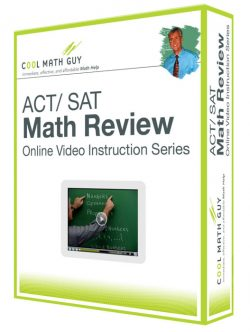# ACT and SAT Math Review

### Course Info

Table of Contents

Introduction

Chapter 1 – ARITHMETIC

• 1.1  Terminology, Symbols, and Operations
• 1.2  Digit Value and Divisibility
• 1.3  Consecutive Integers, Remainders, and Even and Odd Integers
• 1.4  Prime Numbers, Fractions, and Decimals
• 1.5  Percents, Percent Equations, Markup, and Discount
• 1.6  Ratio and Proportion
• 1.7  Average and Distance-Rate-Time
• 1.8  Consumer Problems and Time

Chapter 2 – ALGEBRA

• 2.1  Integers, Terminology, and Operations
• 2.2  Equations: Solving, Substitution, and Systems
• 2.3  More Equations and Word Problems: Age, Work-Rate, Mixture, and Word Interpretation
• 2.4  Exponents and Radicals
• 2.5  Scientific Notation and Inequalities
• 2.6  Plugging In, Factoring, and Solving Equations

Chapter 3 – GEOMETRY

• 3.1  Angles and Parallel Lines
• 3.2  Triangles
• 3.3  Special Right Triangles
• 3.4  Composite Figures
• 3.5  Perimeter, Circumference, and Area
• 3.6  More Problems Involving Circles
• 3.7  Volume
• 3.8  Graphing

Chapter 4 – MISC. TOPICS

• 4.1  Sequences and Number Theory
• 4.2  Counting Principles and Probability
• 4.3  Statistical Averages
• 4.4  Graphs and Statistical Word Play
• 4.5  More Number Theory and Geometric Word Play

Chapter 5 – ADVANCED TOPICS

• 5.1  Absolute Value
• 5.2  Exponents and Radicals
• 5.3  Equations
• 5.4  Slope
• 5.5  Equations of Lines
• 5.6  Functions
• 5.7  Transformations
• 5.8  Rational Expressions and Rational Equations
• 5.9  Distance and Midpoint
• 5.10  Variation
• 5.11  Sets
• 5.12  Sequences
• 5.13  Mean, Median, and Mode
• 5.14  Misc. Geometry
• 5.15  Trigonometry

Course Access Options

•Select options

### ACT / SAT Math Review

From: \$19.95 / month with 1 day free trial
•Add to shopping bag

\$25.00